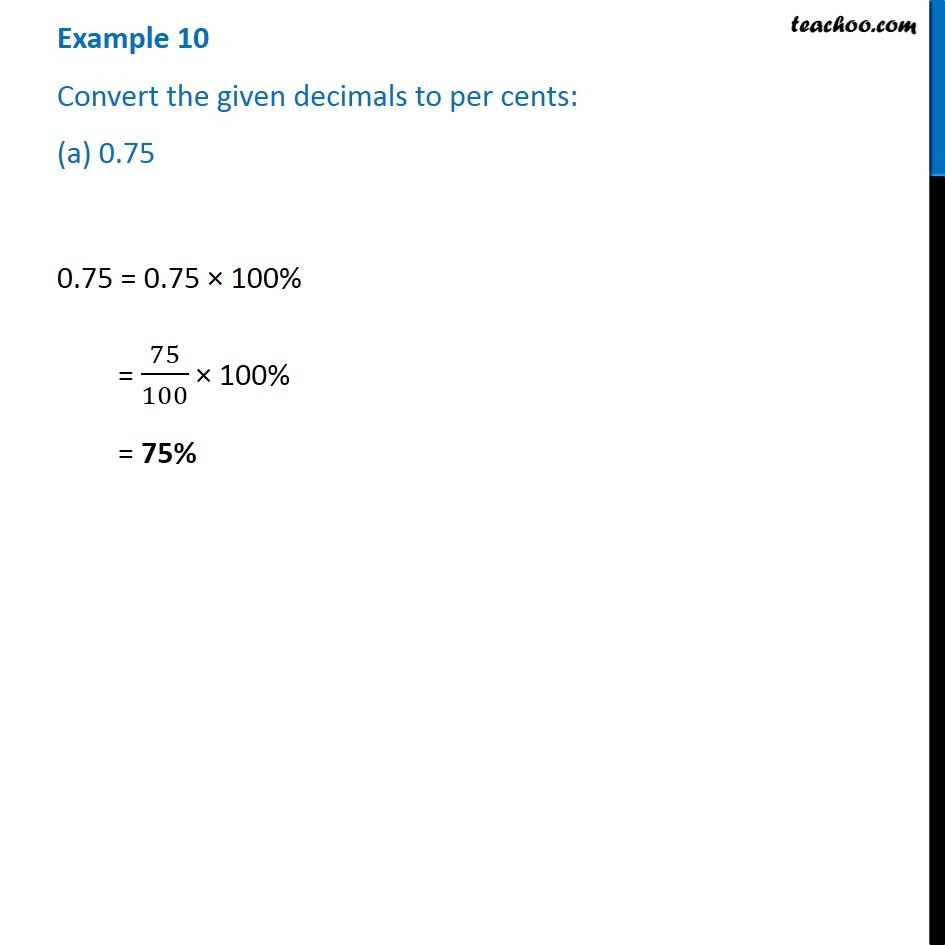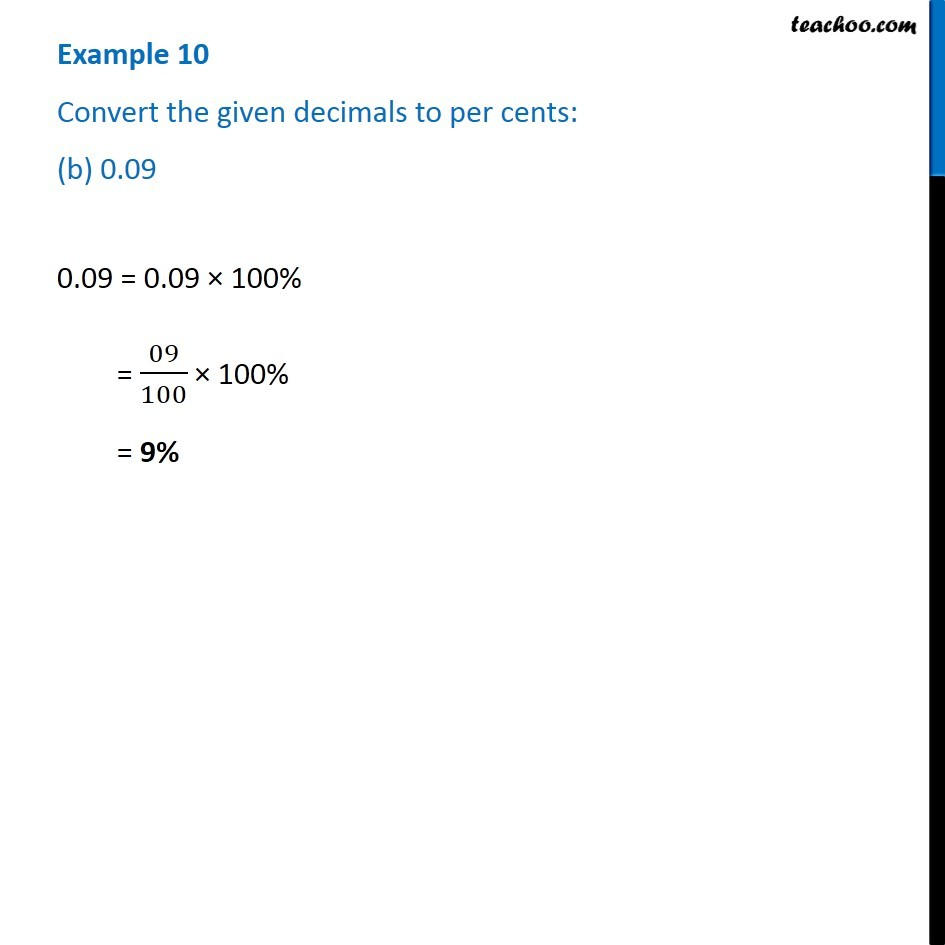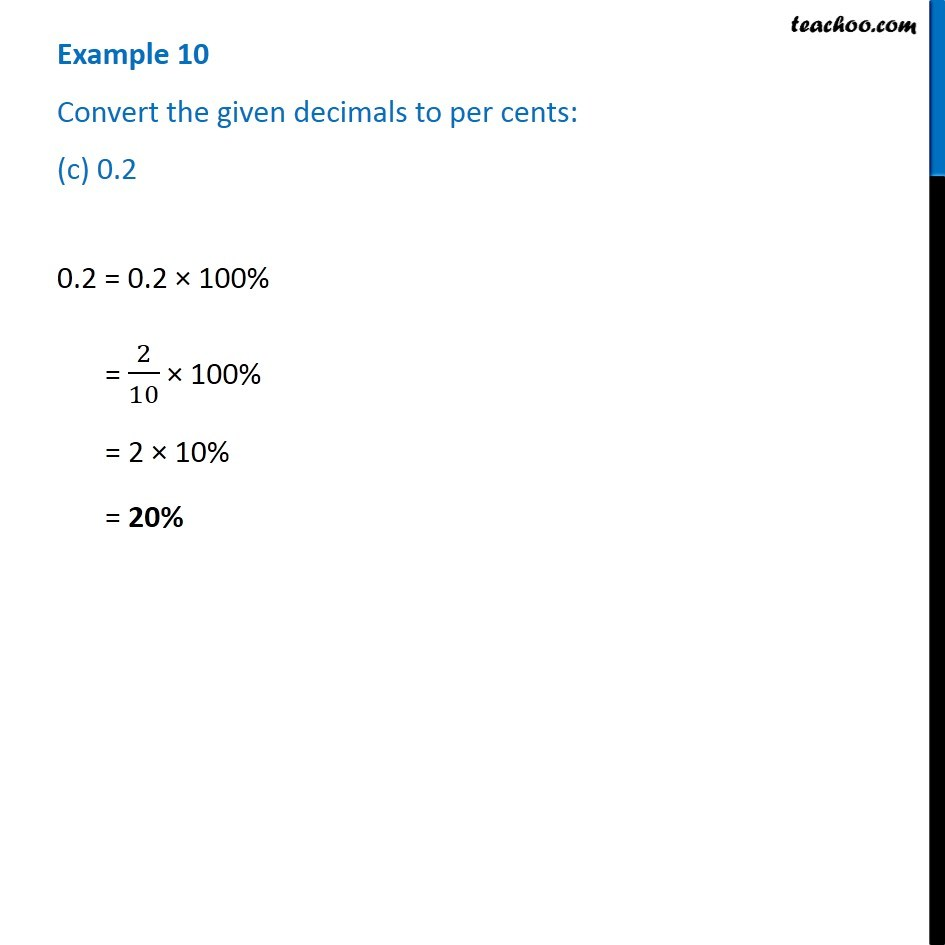Subscribe to our Youtube Channel - https://you.tube/teachoo

1. Chapter 8 Class 7 Comparing Quantities
2. Serial order wise
3. Examples

Transcript

Example 10 Convert the given decimals to per cents: (a) 0.75 0.75 = 0.75 × 100% = 75/100 × 100% = 75% Example 10 Convert the given decimals to per cents: (b) 0.09 0.09 = 0.09 × 100% = 09/100 × 100% = 9% Example 10 Convert the given decimals to per cents: (c) 0.2 0.2 = 0.2 × 100% = 2/10 × 100% = 2 × 10% = 20%

Examples